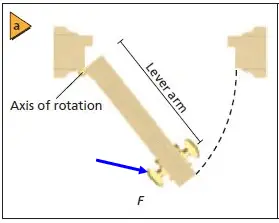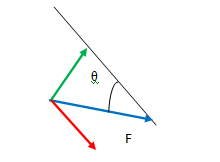# Torque Derivation | derivation of torque

In this article, we will focus on the derivation of Torque with practical examples and diagrams.
1>
– We will derive it with the understanding that it is the moment of force.
2>
– Also, we will show you how to derive the formula of torque, with the understanding that torque is the rate of change of angular momentum (See the analogy: force is the rate of change of linear momentum) and
3>
– the last one, this version of the formula for torque will show torque as the cross product of Moment of Inertia and Angular Acceleration. (again see the analogy, Force is the product of mass and linear acceleration)

The SI unit for torque is the newton metre (N⋅m).

## Torque derivation

As per definition Torque is the moment of force which can be expressed as, t = ±rF
where r is the shortest distance between the rotation axis and the point of application of the force and F ⊥ is the perpendicular component of the force.

While opening a door that is free to rotate about its hinges, the greatest torque is produced when the force is applied farthest from the hinges and at an angle perpendicular to the door. In the diagram below you can find different use cases of this everyday work we do.

Lever arm is the perpendicular distance from the axis of rotation to the point where the force is exerted.

If the force is perpendicular to the radius of rotation, say for the door, it is the distance from the hinges to the point where you exert the force, as illustrated in Figure above.

If the force is not perpendicular, the perpendicular component of the force must be found.The force is not perpendicular here. See the diagram below for resolved components of force FThe effective force that generates torque = Vertically resolved component (in green) of F = F sinθ

From the above diagram, we can see that as the F (in blue arrow) is applied at an angle theta with the lever arm(black line), the entire force F can’t act to create the rotation.

Rather, the effective force that generates torque = Vertically resolved component of F = F sinθ [The component in green in the above diagram]

So we get Torque = T = r F sinθ = r x F ………………(a)

So we can write the expression of torque as the cross product of lever arm length r and the applied force F.

This is the formula we use to do Torque Calculation.

Note for quick revision:
Lever arm length r is the shortest distance between the rotation axis and the point of application of the force and θ = angle made between Lever arm and the line of action of the force being applied.

## Derivation of torque (2 more ways)

We will derive the τ equation or τ formula in 2 different ways.

1. First one as the rate of change of angular momentum

2. And the second one as the moment of Force.

## Torque equation derivation – Rate of change of Angular Momentum with Time

Rate of change of Angular Momentum with respect to time = ΔL/ΔT

Now,  ΔL/ΔT = Δ(I ω)/ΔT = I. Δω/ΔT  ……. (1) [ Here I is constant when mass and shape of the object are unchanged]

Now Δω/ΔT is the rate of change of angular velocity with time i.e. angular acceleration (α).

So from the equation (1), we can write, ΔL/ΔT = I α …………………(2)

Have you noticed this expression I α?

I (moment of inertia) is the rotational equivalent of mass(inertia) of linear motion.
Similarly angular acceleration α (alpha) is the rotational motion equivalent of linear acceleration.

Product of mass m and linear acceleration a represents Force in linear motion.

Similarly I and α in product form represent Torque (τ), which in turn is the rotational equivalent of Force.

So from equation (2) we get, ΔL/ΔT = τ …………….(b)

which states that the rate of change of angular momentum with time is called Torque.

This is one τ equation.

This represents the relation between angular momentum and torque.

## Derivation of Torque formula – torque as the moment of Force

In this post, we have seen that Torque (T) is the moment of force. Τ = r X F = r F sinθ …..(equation #a above)

[ F is the force Vector and r is the position vector (a vector from the origin of the defined coordinate system to the point where the force is being applied).

θ is the angle between the force vector and the lever arm vector. X denotes cross product. ]

Now expanding this by putting F = ma  we get:   (m = mass of the object, and a = linear acceleration)

Torque Τ = r F sin θ = r ma sinθ = r m αr sinθ = mr^2. α sinθ = I α sinθ

T= I X α    ………………(c)
This is another τ equation.

[α (alpha) is angular acceleration, I is moment of inertia and X denotes cross product.]
So τ is cross product of I and α (alpha).

## FAQ

1>Why is torque known as the moment of force?

Answer: Torque is the measure of how effectively a force can twist or turn something. That’s why torque is also known as Moment of Force. (Rotational domain equivalent of Force). Torque is also defined as the rate of change of angular momentum. If the net torque is zero then a body will not change its rotational state of motion. Torque is denoted as T or τ (Tao).